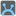• # How not to interpret the prob, and a harder one

## Question related to missionHubspot Amulet

### Background

So it took me a couple of days to solve this problem. It turns out that I had actually misinterpreted the question, and ended up trying to solve one that was significantly harder. So here's the way I should have interpreted it, and the way I did end up interpreting it. If anyone has any insight into the problem I was trying to solve, it was fairly challenging to do in any kind of efficient way, but I would love to know your thoughts. I'll try not to include anything that would be considered too much of a spoiler. If you can adapt what I figured out to apply to the problem as it should be interpreted, well you probably could have solved it anyway.

### How it should be interpreted

When it says that you can only rotate the lever between -180 degrees (to the left) and 180 degrees (to the right), they mean that it can be rotated 180 degrees in either direction relative to its present location. So if you pull first lever 10 degrees, and the second lever turns 20 degrees then you still are able to pull the second lever another 180 degrees in either direction. But the final positions just need to be at [0,225,315] as would be inscribed around the outside of the circle.

### My Interpretation

#### How I interpreted it

When I first looked at this problem, I took it to mean that you could only pull the lever to the position +-180 from zero (That is to the 180 mark). While a brute force solution would work, it very quickly would get out of hand since you're you have to keep computing the valid range for the next rotation after each lever pull. So for the first pull it would be [-180,180], but for the second pull and third pull it would be [-((180 + angle) % 360), (180-angle) % 360] where angle is the absolute position of lever2 on the second pull, and lever3 on the 3rd pull.

#### The Route I took

Soooo, I kinda went down the rabbit hole. Computing the new valid boundaries for each choice of r1, and r2 raises the overhead significantly, and trying to do a linear search for r1 in [-180,180], and r2 and r3 in [-360,360] does so as well. You can precompute boundaries, but you still have to do (%360) after each step to get the absolute position for the next step, so its still problematic. So I started reading up on modular arithmetic and brushing up on linear algebra. If you know modular arithmetic and I'm wrong here, please tell me. If not, then don't learn it from me.

So looking at it from the perspective of a single lever, checking that it gives gives you the final correct position for the lever becomes a problem of solving.

```(rotation_per_degree)*(r1)+0 ≡ a (mod 360)
(rotation_per_degree)*(r2)+a ≡ b (mod 360)
(rotation_per_degree)*(r3)+b ≡ c (mod 360)
```

Where a,b,c are in Z; c is the final position of the lever; and r1,r2,r3 are all valid rotations. Since a,b,c are integers, you can start from one of the equations, manipulate it using the rules for modular arithmetic, and start do some stuff collapsing the equations into one equation.

```1. (rotation_per_degree)*(r1) + 0 ≡ a (mod 360)
2. (rotation_per_degree)*(r1) + 0 + b ≡ a + b (mod 360)
3. (rotation_per_degree)*(r1) + 0 + b + c ≡ a + b + c (mod 360)
```

You can simplify in this way to not have to do a %360 to get rid of all but the final %360 in the 3 equations checking if r1,r2,r3 will rotate onto the final position, but you still would need the itermediate positions to check if the rotations are valid.

But like I said, still couldn't really get it efficient.

#### So on to solving it as a system of simultaneous equations with sympy and numpy

So if you transpose the matrix of coefficients, you can solve for Ax=b, where x is a vector (r1,r2,r3). However, trying to solve a system of linear equations for Ax = b (mod 360), is problematic since it requires alternative strategies of solving the linear system than when solutions are in R^3. Apparently there is some stuff you can do involving reducing the matrix to Hermitage Normal Form, but since the only python library that will do that easily I could find was Sage, that was out. Ultimately, I ended up deciding that however much you pull any of the levers, the lever that turns the most still won't exceed the +- the sum of your 3 largest values in the matrix. So it turned out that it was easiest just to:

1. Find the sum of the 3 highest values in the matrix
2. Take the combinationswithreplacement(range(-thatsum,thatsum+1),3), each of these combinations represent a different number of full rotations that the levers can take
3. Get the permutations of those combinations so that each lever will get each combination
4. Make each of those into a vector
5. Multiply each of those by 360 and add that to the final position
6. Solve each of these
7. Filter out ones that aren't integer solutions or aren't all valid rotations
8. Return the first solution

But yah, that's when I realized that I was solving the wrong problem. Anyways, if you want to look into it, there is some cool other stuff there with residue systems, and something makes me think that the chinese remainder theorem could be useful. But that's enough for now.18